# Math for 10th Grade: Number Systems

Sale ends in
Teacher: Joseph
Customers Who Have Viewed This Course: 580
\$100.00
\$31.00

#### 0 Module Introduction 04:51

This video explains what the course is about, how the videos and materials are arranged, special emphases of the module, and who might benefit from taking the course.

#### 1 What is a Number System, anyway? 08:54

This is a video introduction to the concept of a number system.

#### 2 Lesson 1: Rational and Irrational Numbers 16:42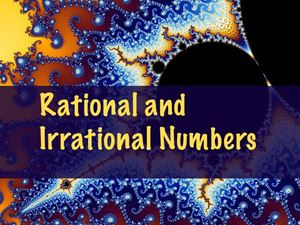Students will distinguish rational numbers as those that can be written in the form of p/q, where p and q are integers.

#### 3 Lesson 1: Answers - Rational and Irrational Numbers 20:19

Answers to problems teaching students to distinguish rational numbers as those that can be written in the form p/q, where p and q are integers.

#### 4 Lesson 2: Addition and Subtraction of Rational Expressions 22:44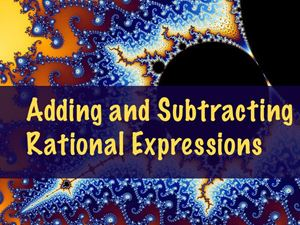Students will extend the rules of operations with common fractions to addition and subtraction of rational polynomial expressions.

#### 5 Lesson 2: Answers - Addition and Subtraction of Rational Expressions 24:57

Answers to the problems set on addition and subtraction of rational numbers and expressions.

#### 6 Lesson 3: Multiplication and Division of Rational Expressions 12:47Students will extend the operations of multiplication and division of common fractions to these operations with ratios of general algebraic expressions.

#### 7 Lesson 3: Answers Part B - Multiplication and Division of Rational Expressions 14:46

Video answers, continued, to the problem set on multiplying and dividing rational expressions.

#### 8 Lesson 3: Answers Part A - Multiplication and Division of Rational Expressions 15:50

Video answers for the problem set on multiplication and division of rational expressions.

#### 9 Lesson 4: Rationalizing the Denominator 18:39Students will apply properties of radicals (roots or surds). Students will use the properties of square roots to rewrite fractions with irrational denominators in standard form.

#### 10 Lesson 4: Answers Part A - Rationalizing the Denominator 14:30

Video answers for problem set on rewriting rational expressions with roots in the denominator in converted form, with rational denominators.

#### 11 Lesson 4: Answers Part B - Rationalizing the Denominator 16:47

Video answers, continued, to problem set on rationalizing the denominator

#### 12 Lesson 5: Part A - Intro to Complex Numbers 15:06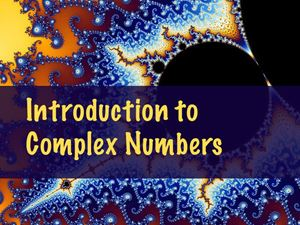Background information on the meaning of the imaginary unit i and the definition of complex numbers as those of the form a + bi, with a real number part a and an imaginary component bi.

#### 13 Lesson 5: Part B - Addition and Subtraction of Complex Numbers 15:46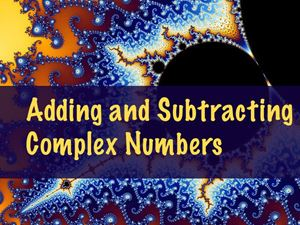Students will add and subtract complex number by combining like terms: the real portion and the imaginary portion of the complex numbers.

#### 14 Lesson 5: Answers - Addition and Subtraction of Complex Numbers 23:10

Video answers to the problem set on addition and subtraction of complex numbers

#### 15 Lesson 6: Multiplication and Division of Complex Numbers 23:20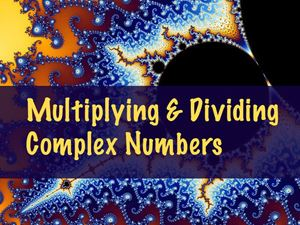Students will multiply complex numbers by applying the multiplication of binomials algorithms, both by general distribution and using an area model. Students will multiply complex conjugates and use them to divide complex numbers.

#### 16 Lesson 6: Answers Part A - Multiplication and Division of Complex Numbers 16:00

Video explanation of problem set on the multiplication and division of complex numbers.

#### 17 Lesson 6: Answers Part B - Multiplication and Division of Complex Numbers 18:02

Answer video, continued, on multiplication and division of complex numbers.

#### 18 Lesson 7: The Complex Plane: Rectangular and Polar Coordinates 26:57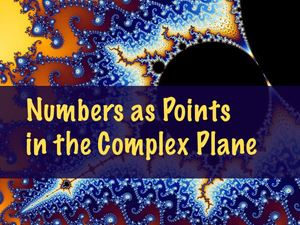Students will represent complex numbers as a coordinate and associated vector in the 2-dimensional plane with a real valued x-axis an imaginary valued y-axis. Students will compute magnitude and argument (direction) of complex numbers.

#### 19 Lesson 7: Answers - The Complex Plane 24:23

Video review of the problem set on how to represent complex numbers as locations on the complex plane in rectangular or polar coordinate systems.

#### 20 Lesson 8: Part A: Operations on Numbers in the Complex Plane 19:48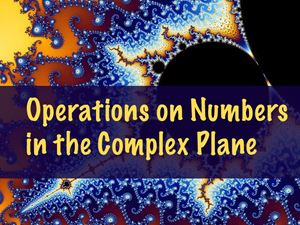Students will add complex numbers represented as vectors in the complex plane. Students will subtract complex numbers by addition of opposites. Students will explore the geometric effect of multiplication by the imaginary unit i.

#### 21 Lesson 8: Part B: Addition, Subtraction, Multiplication in the Complex Plane 16:34

Students will continue to explore operations on complex numbers represented as vectors in the complex plane. Students will determine the effect of multiplication by a scalar and of multiplication by a complex number in the complex plane.

#### 22 Lesson 8: Answers Part A - Operations in the Complex Plane 16:51

Answer video for the problem set on addition, subtraction, and multiplication of numbers represented as vectors in the complex plane.

#### 23 Lesson 8: Answers Part B - Operations in the Complex Plane 24:13

video answers, continued, for problem set of addition, subtraction, and multiplication of numbers represented as vectors in the complex plane

#### 24 Closure 03:35

This wrap-up video brings closure to the module but also explains the mathematical idea of closure. The complex number system is closed under all the algebraic operations and thus exhibits closure. This is what guarantees, via the Fundamental Theorem of Algebra, the existence of exactly n roots for a single-variable polynomial of degree n.

Course Overview

This "10th Grade Math: Number Systems" module is a foundational module for understanding how to work with all the number systems encountered in algebraic and geometric computation at the high school level. It reinforces the theory of computation with fractions and extends that to variable expressions in rational form. The module deals extensively with conceptual understanding of square roots and how to simplify them and manipulate expressions containing square roots without relying on technology. The module culminates with an explanation of complex numbers in various representations and emphasizes the utility of complex numbers.

The focus of this course is on conceptual understanding and reasoning, not memorization or routine calculation. Problems will be embedded in real contexts. The exercise sets are designed to become progressively more challenging so that completion of the problems will generate new learning and not just check for understanding.

This module includes

• 8 LESSONS IN 10 VIDEOS

• 8 PROBLEM SETS WITH COUNTERPART ANSWER SHEETS

• OVER 135 PRACTICE EXERCISES/PROBLEMS

• 12 EXERCISE REVIEW VIDEOS (with step-by-step explanation how to reach an answer and suggested alternate approaches)

• A CUMULATIVE UNIT EXAM (40+ QUESTIONS) IN 2 PARTS WITH COUNTERPART ANSWER KEY AND SOLUTIONS

• JUST UNDER 7 HOURS OF VIDEO-LESSONS

Course Goals

Upon completion of this course, students will demonstrate facility with systems of numbers beyond simple integer and decimal values. Students will be able to distinguish and classify integers, rational numbers, irrational numbers, real numbers, imaginary numbers, and complex numbers. Students will master the properties and operations of fractions as rational numbers and be able to extend these properties to rational expressions including variable and polynomial expressions.

Students will be able to identify the properties of irrational numbers and formalize the rules for working with square roots of positive real numbers. Students will be able to multiply roots and provide solutions to equations in simplified radical form. Students will apply the use of square roots in common geometric contexts involving rectangles and right triangles, especially the isosceles right triangle, the 30-60-90 right triangle, and Pythagorean triples. Students will also be able to apply the rules of multiplying roots to rationalize the denominator of rational expressions.

Students will develop the concept of the imaginary number i and the set of imaginary and complex numbers. Students will be able to add and subtract complex numbers as algebraic expressions. Students will also multiply complex numbers, use complex conjugates to rationalize expressions with complex denominators, and divide by complex numbers. Students will be able to represent complex numbers as points in the complex plane with a real axis and imaginary axis, in both rectangular and polar forms. Students will be use vectors to represent complex numbers in the plane in order to add, subtract, and multiply complex numbers. Students will be able to compute the magnitude (absolute value or length) of a number in the complex plane and the argument (directional angle of rotation) of a complex number. These explorations will include a brief application of the tangent of an angle, an introduction to the Fundamental Theorem of Algebra and the existence of complex roots, and connections to real-world applications in alternating current circuit design.

Target Audience

This video-course is primarily intended as a foundational module for a full course in 10th grade mathematics in an integrated algebraic and geometric approach. However, it is appropriate for students in 8th-11th grade in any algebra-intensive course.

Course Requirements

Students taking this module will need to have completed some previous algebra. This module assumes facility using the Pythagorean Theorem, the ability to multiply binomial expressions, and previous experience factoring quadratics. Experience with special right triangles will be helpful to the student. Students need to be able to locate points in the coordinate plane.

Course Topics

Lesson 1: Rational and Irrational Numbers

Understand that rational numbers can be expressed in the form p/q, where p and q are members of Z, the integers.

Distinguish rational and irrational numbers

Express numbers in the form p/q, if rational.

Use set notation to demonstrate the hierarchy of integers (Z), rationals (Q), and irrationals (Q') within the real numbers (R).

Lesson 2: Add and Subtract Rational Expressions

Add and subtract simple common fractions

Adding rational expressions with a common denominator

Add and subtract rational expressions with denominators that are relatively prime

Add and subtract rationa expressions with denominators that share a common factor

Lesson 3: Multiply and Divide Rational Expressions

Division and multiplication as related operations

Multiplying rational expressions

Dividing rational expressions by inverting the divisor

Undefined expressions and division by zero

Restricted domains

Lesson 4: Rationalizing the Denominator

Definition of the square root, properties of square roots defined

Rationalizing the denominator by multiplying a simple root

Applications to geometry and special right triangles

Rationalizing the denominator by multiplying by the conjugate

Lesson 5: Introduction to Complex Numbers and Adding and Subtracting Complex Numbers

Defining the imaginary number i

Constructing complex numbers in the form a + bi

The hierarchy of sets of numbers including real, imaginary, and complex numbers

Review: Identifying numbers by set: integer (Z), rational (Q), irrational (Q'), real (R), imaginary (I), or complex (C)

Adding and subtracting complex numbers by combining like terms (horizontally or vertically)

Applications (RLC series circuits)

Lesson 6: Multiplying and Dividing Complex Numbers

Repeated multiplication by i

Squaring terms with i

Multiplying a complex number by a scalar

Multiplying a complex number by a complex number with various models

Multiplying complex conjugates

Using the complex conjugate to divide complex numbers

Applilcations (RLC parallel circuits)

Lesson 7: Complex Numbers Represented as Points in the Complex Plane

The real and imaginary axes

Points in the rectangular coordinate system as complex numbers

Complex numbers as vectors in the plane

The magnitude (absolute value) of a complex number, |z|, as the length of the vector

The argument (direction) of a complex number as the angular rotation of the vector

Points in the polar coordinate system as complex numbers

Lesson 8: Operations with Complex Numbers in the Complex Plane

Subtracting complex numbers by adding inverse vectors

Multiplication by i as a 90° rotation about the origin

Scalar multiplication as a dilation of the vector

Multiplying two complex vectors: the effects on both magnitude and direction

Converting from polar form to rectangular form

Solving roots of unity, plotting the solutions, and the Fundamental Theorem of Algebra

Common Core State Standards Alignment

This module directly, but not exhaustively, addresses many of the standards in the CCSS Math High School: Number and Quantity category.

According to the CCSS, much of the content on rational and irrational numbers should have been mastered in the middle grades. However, many high school students need further exposure to and practice with rational and irrational numbers. Conceptual understanding of fractions (rational numbers) is often lacking, and computation with irrational numbers (especially square roots) is often strictly calculator-based. This module revisits those standards with more emphasis on understanding than use of rote algorithms or the calculator as the source of answers.

These standards relating to complex numbers receive significant emphasis:

CCSS-Math-HS Numbers and Quantity: The Complex Number System:

A1: Know there is a complex number i such that i2 = -1, and every complex number has the form a + bi with a and b real.

A2: Use the relation i2 = -1 and the commutative, associative, and distributive properties to add, subtract, and multiply complex numbers.

A3: Find the conjugate of a complex number; use conjugates to find moduli and quotients of complex numbers.

B4: Represent complex numbers on the complex plane in rectangular and polar form (including real and imaginary numbers), and explain why the rectangular and polar forms of a given complex number represent the same number.

B5: Represent addition, subtraction, multiplication, and conjugation of complex numbers geometrically on the complex plane; use properties of this representation for computation.

C9: Know the Fundamental Theorem of Algebra; show that it is true for quadratic polynomials.

These standards relating to vectors are introduced in relation to complex numbers expressed as vectors in the complex plane:

CCSS-Math-HS Numbers and Quantity: Vector and Matrix Quantities:

A1: Recognize vector quantities as having both magnitude and direction. Represent vector quantities by directed line segments, and use appropriate symbols for vectors and their magnitudes (e.g., v, |v|, ||v||, v).

B5: Multiply a vector by a scalar.

• Teacher: Joseph
• Areas of expertise: NBCT in Secondary Mathematics; Curriculum Design, Advanced Placement Calculus AB and International Baccalaureate Diploma Programme Math Standard Level
• Education: BA in Mathematics, Biology, Secondary Education, Greenville University; MA in Secondary Education, University of Alabama
• Interests: Science Fiction
• Skills: Curriculum development, gardening, volleyball, outdoor education
• Associations: NBCT (National Board of Certified Teachers)
• Issues I care about: Educational opportunity, food security, clean water

I love discovering new things about mathematics, and I enjoy teaching. It is great fun working with excited high school students. Our own two children were homeschooled through high school and are now in college.

Problem set for this lesson with the answers included. Some solution steps are included for some problems, to accompany the full solutions in the answer video.

Problem set for this lesson with the answers included. Some solution steps are included for some problems, to accompany the full solutions in the answer video.

Problem set for this lesson with the answers included. Some solution steps are included for some problems, to accompany the full solutions in the answer video.

Problem set for this lesson with the answers included. Some solution steps are included for some problems, to accompany the full solutions in the answer video.

Problem set for this lesson with the answers included. Some solution steps are included for some problems, to accompany the full solutions in the answer video.

Problem set for this lesson with the answers included. Some solution steps are included for some problems, to accompany the full solutions in the answer video.

Problem set for this lesson with the answers included. Some solution steps are included for some problems, to accompany the full solutions in the answer video.